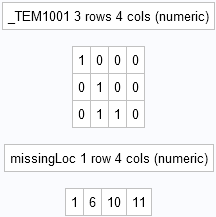A common task in SAS/IML programming is finding elements of a SAS/IML matrix that satisfy a logical expression. For example, you might need to know which matrix elements are missing, are negative, or are divisible by 2.

In the DATA step, you can use the WHERE clause to subset data. You can also use IF-THEN/ELSE logic to examine each observation in a data set to determine whether it satisfies a criterion. For data that are stored in a SAS/IML matrix, some programmers write a loop that iterates over the rows and/or columns and use IF-THEN/ELSE logic on each scalar element in the matrix. However, there is an easier and more efficient way: Use the LOC function, which finds the LOCation of elements that satisfy a logical expression.

This article provides examples of using the LOC function and a logical expression to find elements in a matrix that satisfy a criterion. It also points out that the SAS/IML language creates temporary 0/1 matrices from logical expressions.

The following SAS/IML statements define a 3 x 4 matrix. The LOC function is used to find the location of the elements that are missing, negative, and even, respectively:

```proc iml; x = {. -5 2 5, -2 . 3 4, 4 . . -1}; missingLoc = loc(x=.); /* missing values */ negativeLoc = loc(x^=. & x<0); /* nonmissing and negative */ evenLoc = loc(mod(x,2)=0); /* n is even if (n mod 2)=0 */ print missingLoc, negativeLoc, evenLoc;```The output shows the location of the elements for each criterion. The elements of the matrix are enumerated in row-major order: the first row contains elements 1–4, the second row contains elements 5–8, and the third row contains elements 9–1. The program output shows that missing values are stored at locations 1, 6, 10 and 11. Negative values are stored at locations 2, 5, and 12. Even integers occur at locations 3, 5, 8, and 9.

Even if you are familiar with the LOC function, you might not be familiar with the way SAS/IML passes a logical expression like x=. or x<0 into the LOC function. When the SAS/IML parser sees an expression that involves a comparison operator, it evaluates the logical expression, which creates a temporary matrix of 0s and 1s. That temporary 0/1 matrix is sent to the LOC function. You can observe this behavior by using the RESET PRINTALL statement to display all matrices that SAS/IML creates, including the temporary matrices. If you add the statement

`reset printall;`

as the second line of the previous program, then the following output is displayed when the first LOC function executes:The output shows that PROC IML creates a temporary matrix named _TEM1001. The matrix is the result of evaluating the logical expression x=.. Notice that the 0/1 matrix contains a 1 in the locations for which the data are missing and a 0 in the other locations. Similarly, the other logical expressions in the program create temporary matrices with names like _TEM1002 and _TEM1003.

There are two takeaways from this example:

• Use the LOC function to find the location of matrix elements that satisfy a logical condition. The LOC function is more efficient than an explicit loop over the matrix elements.
• The argument that is passed to the LOC function is always a matrix. When you specify a logical condition, SAS/IML creates temporary variables from the logical expressions. You can use the RESET PRINTALL statement to see the temporary variables that are created.

ShareDistinguished Researcher in Computational Statistics

Rick Wicklin, PhD, is a distinguished researcher in computational statistics at SAS and is a principal developer of SAS/IML software. His areas of expertise include computational statistics, simulation, statistical graphics, and modern methods in statistical data analysis. Rick is author of the books Statistical Programming with SAS/IML Software and Simulating Data with SAS.

1.Hello Rick,
temp1=(temp/x)##x;/*temp1 is a raw vector*/
tempexp=exp(-temp1);/*tempexp is a raw vector*/
tempexpsub=d1row-tempexp;/*tempexpsub is a raw vector results from subtract temexp from d1row (which is a raw vector of one's)*/
templog=log(tempexpsub);
during the calculations, sometimes there is an error for templog. I think , the program approximated the value o temexpsub when it is very small to 0 which causes problem to calculate the log.

I need to know how I can make SAS uses the actual results from tempexpsub matrix without any approximations or truncation of the values of this matrix to be able to calculate the log( tempexpsub) when the values of temexpsub are very small such that they close to zero?

Thank you

•I suggest you post your question to the SAS/IML Support Community, which is the appropriate place for questions that are not directly related to blog posts.

2.Thanks for your response. I can summarize my question in the following point. If the program contains matrix work, I need to know the command to write at the beginning of the program to use the results themselves of a part of the program in another part. I mean if the resulted number is 0.0000000001, then it is used without approximating it to 0. So there will not be a problem to use the log function for the elements of the final matrix.

Thank you